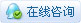2135浏览

# [ 虚谷号入门] 【虚谷号】“石头剪刀布”模型# 1. 原理介绍# 2. 准备工作

### 1）环境搭建。

``````pip install keras

pip install -i https://pypi.tuna.tsinghua.edu.cn/simple tensorflow``````

### 2）数据准备和清洗

``````::
cd ~/Jupyter/vvBoardBook/5.机器学习/data tar -xf hand.tar``````

``````#遍历文件夹中图片的函数
import os
image_types = (".jpg", ".jpeg", ".png", ".bmp", ".tif", ".tiff")
def list_images(basePath, contains=None):
# 返回有效的图片路径数据集
return list_files(basePath, validExts=image_types, contains=contains)
def list_files(basePath, validExts=None, contains=None):
# 遍历图片数据目录，生成每张图片的路径
for (rootDir, dirNames, filenames) in os.walk(basePath):
# 循环遍历当前目录中的文件名
for filename in filenames:
# if the contains string is not none and the filename does not contain
# the supplied string, then ignore the file
# 无意中发现mac处理的文件夹会生成“._”开头的文件，于是过滤
if contains is not None and filename.find(contains) == -1 or filename[0:2]=='._':
continue
# 通过确定.的位置，从而确定当前文件的文件扩展名
ext = filename[filename.rfind("."):].lower()
# 检查文件是否为图像，是否应进行处理
if validExts is None or ext.endswith(validExts):
# 构造图像路径
imagePath = os.path.join(rootDir, filename)
yield imagePath

#定义标签对应的数字，石头为0，剪刀为1，布为2
label_num=["rock","scissors","paper"]
#得到训练文件夹中的图片文件列表
rps_Paths = sorted(list(list_images('./data/hand/rps/')))
#得到验证文件夹中的图片文件列表
test_Paths = sorted(list(list_images('./data/hand/rps-test-set/')))``````

``````from keras.preprocessing import image
import numpy as np
# 生成训练集数据
rps_data = []
rps_labels = []
for imagePath in rps_Paths:
r_img = image.img_to_array(r_img,dtype="uint8")
rps_data.append(r_img)
# 读取标签，并且用0，1，2来编码
#(在“./dataset/rps-test-set/paper/testpaper01-00.png”中取出“paper”)
t = imagePath.split(os.path.sep)[-2]
# 将“paper”之类的单词转换为0，1，2
rps_labels.append(label_num.index(t))
rps_data = np.array(rps_data)
rps_labels = np.array(rps_labels)
# 生成验证集数据
test_data = []
test_labels = []
for imagePath in test_Paths:
r_img = image.img_to_array(r_img,dtype="uint8")
test_data.append(r_img)
t = imagePath.split(os.path.sep)[-2]
test_labels.append(label_num.index(t))
test_data = np.array(test_data)
test_labels = np.array(test_labels)``````

# 3. 训练过程

### 1）搭建模型

``````import keras
from keras import layers
from keras import datasets
model=keras.models.Sequential()
#在训练过程中打印出准确率（acc）指标

### 2)训练模型

``model.fit(x=rps_data,y=rps_labels,batch_size=200,epochs=5,validation_data=(test_data,test_labels))``

batch_size设置为200的情况下，前三轮loss一直处于10左右，第四轮开始，loss快速下降。10个轮次后，就loss就到了0.01以下。每一轮次需要95秒，还是能够接受的。### 3)测试模型

``````from keras.preprocessing import image
import numpy as np
import cv2
#基本函数1：获取摄像头内容保存为图片
def getcampic(fname):
cap = cv2.VideoCapture(0)        # 打开摄像头
ret, frame = cap.read()       # 读摄像头
cv2.imwrite(fname,frame)
cap.release()
cv2.destroyAllWindows()
return True
##调用getcampic函数
getcampic("test.jpg")
label_name=["rock","scissors","paper"]
i_data=[]
r_img = image.img_to_array(r_img,dtype="uint8")
i_data.append(r_img)
i_data = np.array(i_data)
t=model.predict_classes(i_data)
#直接输出名称
print(label_name[t])``````# 5. 参考资料

### 1.从神经网络到卷积神经网络（CNN）

https://www.jianshu.com/p/1ea2949c0056

### 2.CNN（卷积神经网络）是什么？

https://www.zhihu.com/question/52668301

 您需要登录后才可以回帖 登录 | 立即注册 本版积分规则 回帖并转播 回帖后跳转到最后一页

[[wsData.name]]

#### 硬件清单

• [[d.name]]#### 楼主的其它帖子